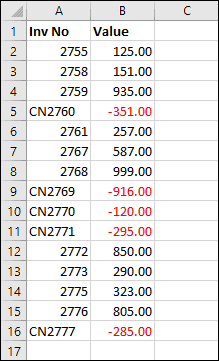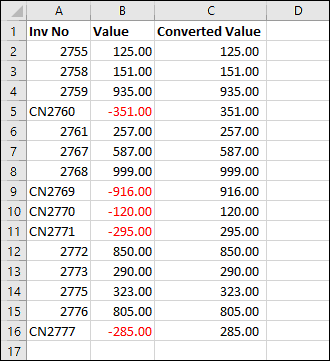# Convert negative numbers to positive in Excel

## Simple conversion of negative numbers into positive numbers in Excel

Simply converting a negative number into a positive number is easy. All you need to do is multiply it by -1 with a formula like this:

=A1*-1

If Excel formulas are unfamiliar to you, you could benefit greatly from our completely free Basic Skills E-book.

Sometimes, however, your needs are more complex than this.

## Converting negatives into positives without converting positives to negatives

Consider the data on the right.

In this example data, invoices are shown as positive values and credit notes are shown as negatives. This is usually the way you want the data to be displayed, but you might want to convert all of these values into positives for presentation purposes, or to make it easier to summarize in a pivot table.

If you were to multiply all of the values in column B by -1 you would succeed in converting the negative values into positives, but you’d also convert all of the positive values into negatives!

The solution is the ABS function. ABS (or Absolute) converts negative values into positives and leaves already-positive values alone.

You could also solve this problem using the IF function, but ABS is a much easier option.

Advanced functions such as IF are covered in depth in our Expert Skills Books and E-books.You can download an example workbook showing the ABS function in action.Scroll to Top